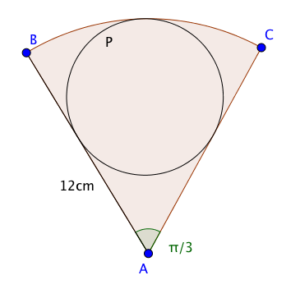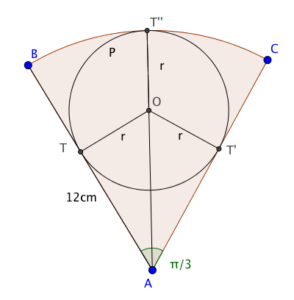Dear Uncle Colin,I got stuck on this sector question, which asks for the radius of circle $P$, which touches sector $ABC$ as shown. I’m given that $ABC$ is a sector of a circle with centre $A$ with radius 12cm, and that angle $BAC$ is $\frac{\pi}{3}$. My answer was 3.8cm, but apparently it should be 4cm. - Someone Explain Circles To Otherwise Reasonable Student

Hi, SECTORS, and thanks for your message!

The answer is indeed 4cm, and here’s how you can work it out.I started by drawing radii from the tangent points on AB and AC to the centre of the circle, and the line of symmetry of the sector. This gives us a radius to each tangency point - but it also gives us two right-angled triangles.

ATO is right-angled at T, and has an angle of $\frac{\pi}{6}$ at A, by symmetry. That means (because $\sin\left(\frac{\pi}{6}\right)=\frac{1}{2}$), $OA=2OT=2r$.

Aha! That means line segment $AT”=3r=12$cm (because it’s a sector of a circle) and $r=4$cm as a result.

Hope that helps!

- Uncle Colin

* Updated 2017-06-28 to fix a typo. Thanks to @singinghedgehog for the correction.